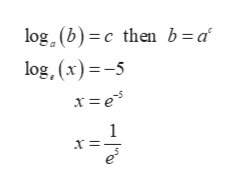Use the definition of the logarithmic function to find x. (Simplify your answer completely.)(a)    ln(x) = −5(b)    ln(1/e) = x

Question

Use the definition of the logarithmic function to find x. (Simplify your answer completely.)

(a)    ln(x) = −5

(b)    ln(1/e) = x

Step 1

Part (a): ln(x) = - 5

Use the logarithmic def...help_outlineImage Transcriptioncloselog . (b) c then b=a log, (x)-5 x= e 1 х- fullscreen

Want to see the full answer?

See Solution

Want to see this answer and more?

Our solutions are written by experts, many with advanced degrees, and available 24/7

See Solution
Tagged in

Other